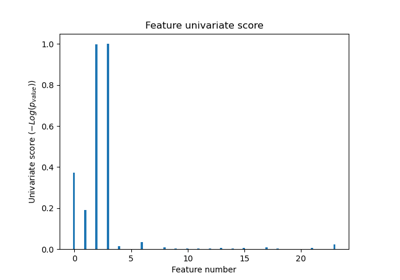# sklearn.feature_selection.f_classif¶

sklearn.feature_selection.f_classif(X, y)[source]

Compute the ANOVA F-value for the provided sample.

Read more in the User Guide.

Parameters: X : {array-like, sparse matrix} shape = [n_samples, n_features] The set of regressors that will be tested sequentially. y : array of shape(n_samples) The data matrix. F : array, shape = [n_features,] The set of F values. pval : array, shape = [n_features,] The set of p-values.

chi2
f_regression
## Examples using sklearn.feature_selection.f_classif¶